﻿

### Open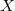$X$-ranks with respect to Segre and Veronese varieties

#### Abstract

Let$X\subset \mathbb{P}^N$ be an integral and non-degenerate variety. Recall (A. Bialynicki-Birula, A. Schinzel, J. Jelisiejew and others) that for any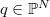$q\in \mathbb{P}^N$ the open rank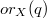$or_X(q)$ is the minimal positive integer such that for each closed set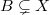$B\subsetneq X$ there is a set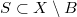$S\subset X\setminus B$ with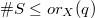$\#S\le or_X(q)$ and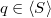$q\in \langle S\rangle$, where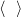$\langle \ \ \rangle$ denotes the linear span. For an arbitrary$X$ we give an upper bound for$or_X(q)$ in terms of the upper bound for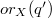$or_X(q')$ when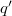$q'$ is a point in the maximal proper secant variety of$X$ and a similar result using only points$q'$ with submaximal border rank. We study$or_X(q)$ when$X$ is a Segre variety (points with$X$-rank$1$ and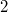$2$) and when$X$ is a Veronese variety (points with$X$-rank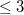$\le 3$ or with border rank$2$).

DOI Code: 10.1285/i15900932v41n1p19

Keywords: open rank; open $X$-rank; Segre variety; Veronese variety; secant variety; border rank

Full Text: PDF